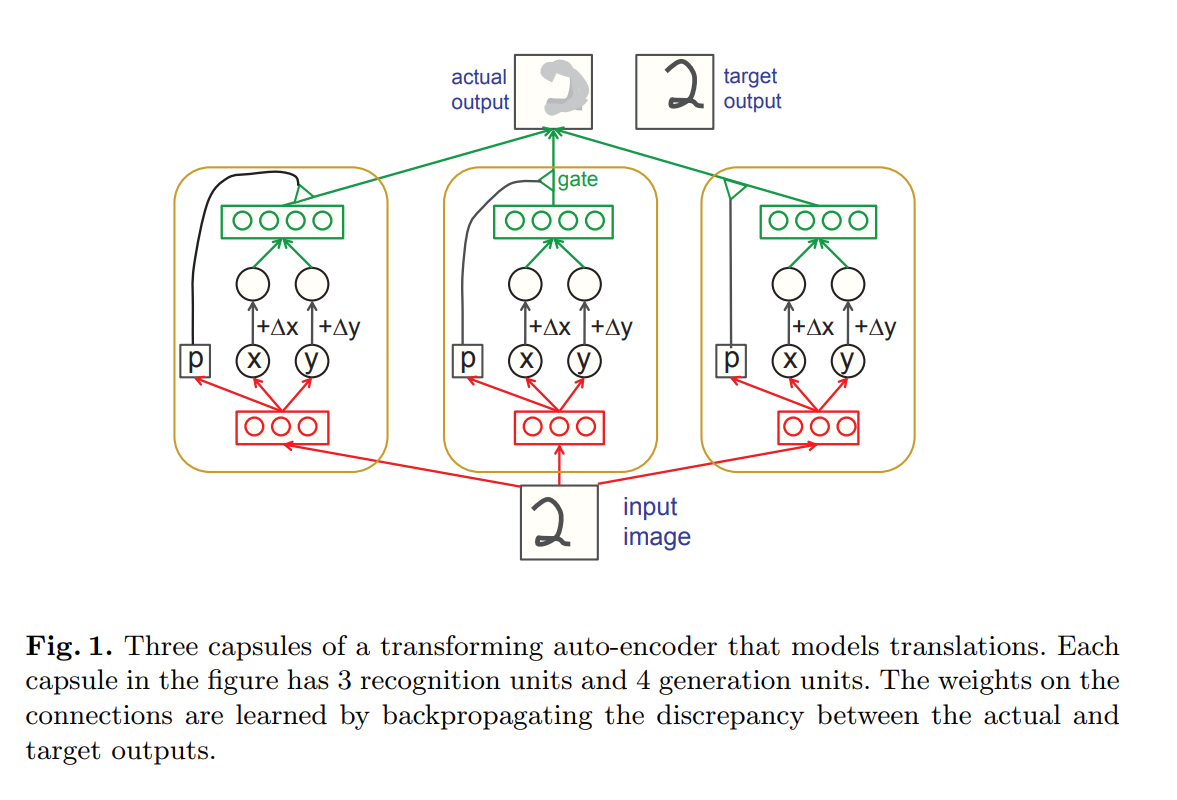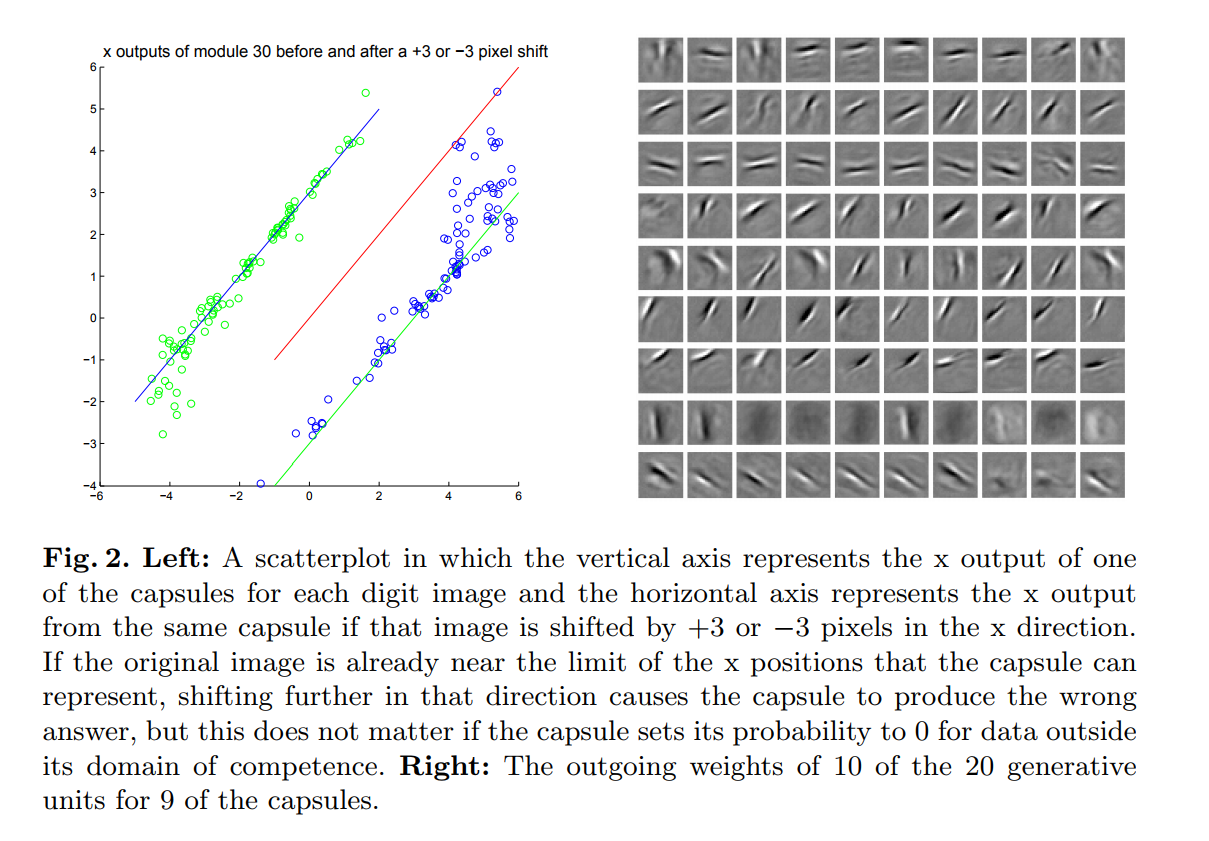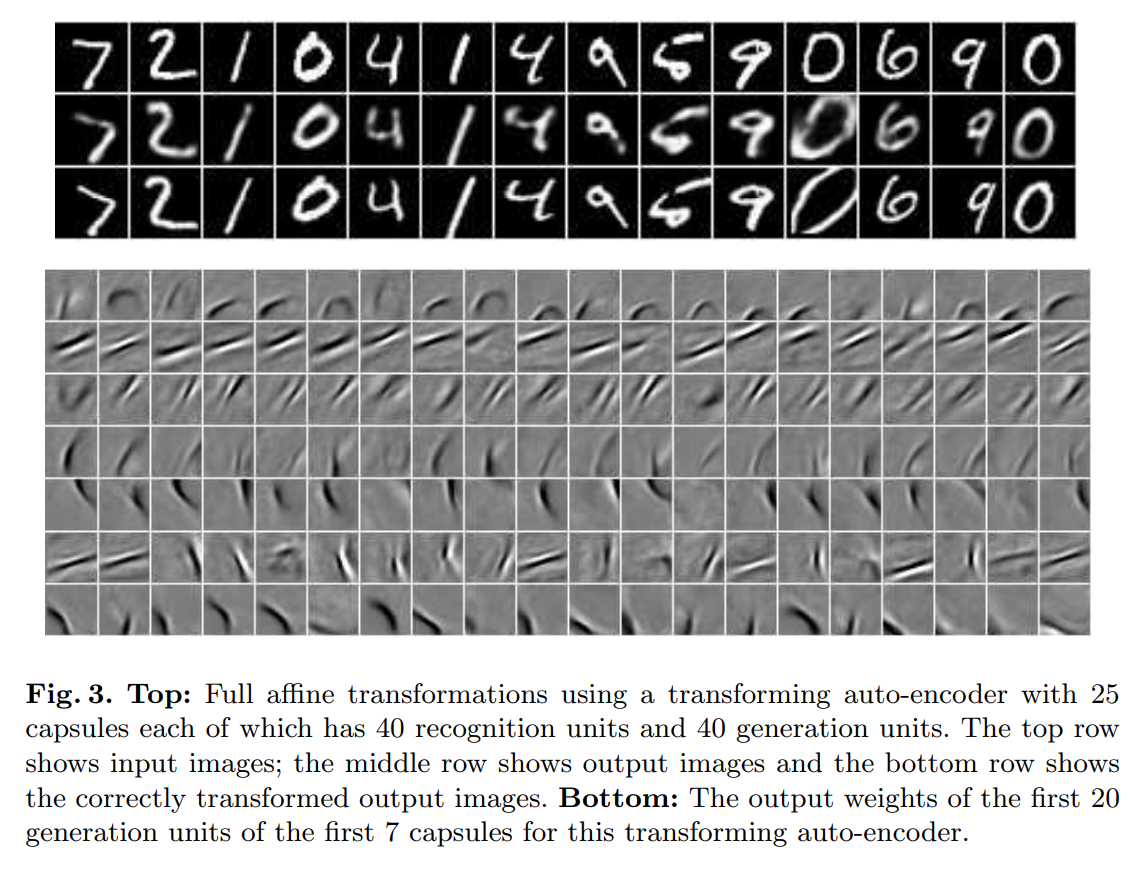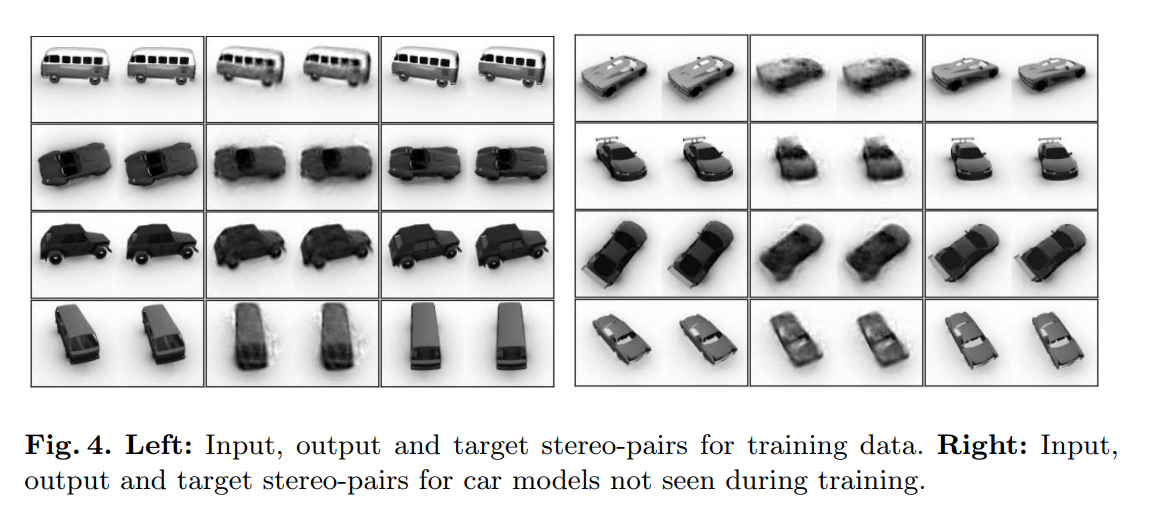## Summary

A network is composed of capsules, each of which learns to recognize and spatially position a visual entity (part of an image). Once the visual entity has been positioned, a transformation can be applied to the coordinates before generating the transformed image.

The network takes as input an image and translations $$\Delta x$$ and $$\Delta y$$. Each capsule is split in two layers; a recognition layer that outputs the $$x$$ and $$y$$ coordinates of its learned visual entity plus a gating value $$p$$ (probability that the entity is present in the image), and a generation layer, which takes (transformed) $$x$$ and $$y$$ coordinates as input, and outputs a “partial” image. The output partial image of each capsule is multiplied by its gating value $$p$$ before being combined with all the other images to produce the final transformed image.Instead of 2D translations, the network can also be used for more complex transformations, or be used in higher dimensions. For example, instead of predicting coordinates, each capsule could predict a $$3 x 3$$ matrix representing a full 2D affine transformation.

## Experiments and Results

Here is an example of how the predicted coordinates of a capsule change when a translation is applied to the input image:Other results:## Issues

A capsule represents a single visual entity, thus the network cannot deal with multiple instances of the same entity.